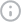0 hidden charges. 0 forex# Understanding Net Asset Value (NAV): Definition, Formula, Uses with ExampleFACT CHECKED
Reviewed by
.
Created on
May 24, 2023## Summary

What’s Inside

Net Asset Value (NAV) is crucial for evaluating mutual funds. It is the value of each fund share and helps investors determine the worth of their investments in the fund. This article will explore the definition, formula, example and uses of NAV for mutual funds.

## Understanding Net Asset Value (NAV)

Net asset value (NAV) is the value of each mutual fund share. It is computed by deducting the fund's liabilities from its assets and dividing the result by the number of outstanding shares. It is the amount investors would receive for each share if they sell the mutual fund at the current market value.

## Calculation of Net Asset Value (NAV) Explained

The Net Asset Value formula is pretty straightforward:

Net Asset Value (NAV) = (Total Asset - Total liabilities) / number of shares

Where:

• Total Asset = market value of all securities held by the mutual fund
• Total liabilities = the amount owned by the mutual fund to creditors

The number of outstanding shares = the total number of shares issued by the mutual fund.

## Illustrating Net Asset Value (NAV) Calculation with an Easy-to-Follow Example

Let's understand net asset value mutual funds with an example:

Let's assume a mutual fund has total assets of INR 10,000,000, total liabilities of INR 2000000, and INR 1000000 outstanding shares. The Net Asset Value for the mutual fund would be:

Net Asset Value = (10000000 - 2000000) / 1000000 = INR 8 per share

## Uses of Net Asset Value (NAV)

The net asset value is a crucial factor that investors use to evaluate the performance of mutual funds. Some of the uses of NAV in investment management include:

1. Measuring the fund's performance - NAV is a reliable metric for measuring the performance of mutual funds. By comparing the NAV of a fund over time, investors can determine whether the fund is generating positive or negative returns. So calculating the net asset value of mutual funds is a primary step in measuring the fund's performance.
2. Buying and selling shares - NAV is the price at which investors buy and sell shares of mutual funds. Investors can use NAV to determine the value of their investment and make informed investment decisions.
3. Fund management - Mutual fund companies use NAV to manage the fund's portfolio. The NAV is used to derive the value of the fund's assets and liabilities and compute the fund's expense ratio.

## Conclusion

Net Asset Value (NAV) is a crucial metric in mutual fund investing. It helps investors determine the value of their investments, evaluate the performance of mutual funds, and make informed investment decisions. The formula to calculate NAV is simple and estimated daily at the end of the trading day. By understanding the concept of NAV and its best uses, investors can make informed investment decisions that align with their financial goals.

Fi, as a money management platform, provides many investment possibilities. It is simple to invest in the short or long term with a single swipe of your phone's screen. Say hello to Fi Money!

### 1. Is Net Asset Value equal to equity?

Net Asset Value (NAV) is not equal to equity. NAV is the total value of assets a mutual fund holds minus any liabilities, divided by the total number of outstanding shares. On the other hand, equity is a company's ownership interest, which represents the residual value of assets after deducting liabilities. In contrast, both NAV and equity can represent the value of a company or a fund. But they both are different and represent distinct concepts.

### 2. Which NAV is good, high or low?

It depends. Generally, a high NAV indicates that the value of the fund's assets has increased. In contrast, a low NAV indicates that the value has decreased. However, more than the net asset value alone is needed to determine whether the fund is good. It is essential to consider other factors such as the performance history, expense ratio, and overall market conditions.

A high Net asset value mutual fund does not necessarily mean it is expensive or will perform better than a fund with a lower NAV. Investors must do thorough research and analysis before investing in a fund.

# DisclaimerInvestment and securities are subject to market risks. Please read all the related documents carefully before investing. The contents of this article are for informational purposes only, and not to be taken as a recommendation to buy or sell securities, mutual funds, or any other financial products.